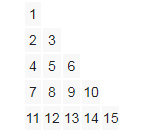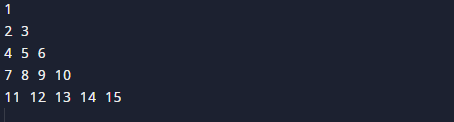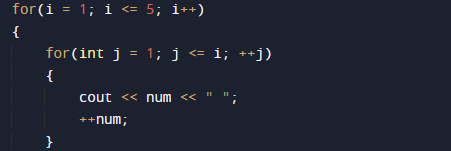# C++ Program to Create Floyd’s Triangle

In this tutorial you will learn about the C++ Program to Create Floyd’s Triangle and its application with practical example.

In this tutorial, we will learn to create a c++ program that will create a Floyd’s Triangle using c++ programming.

## Prerequisites

Before starting with this tutorial we assume that you are best aware of the following C++ programming topics.

• if-else Statements.
• Looping statement.
• Basic input/output.
• Operators.

## What is Floyd’s Triangle ?

It is named after Robert Floyd who created this Triangular shape. Floyd’s triangle is a triangular array of  numbers.It is a method of showing ,filling the rows of the triangle with consecutive numbers, starting with a 1 in the top left corner.## C++ Program to Creating a Floyd’s Triangle

In this program we will print Floyd’s Triangle using nested  for loop. We would first declared and initialized the required variables. Then we will create the Pyramid using below program.

## OutputIn the above program, we have first declared and initialized a set variables required in the program.

• i & j= for  iteration.

In above program  first we declare variables i and j, here we use 2 for loops.The outer loop will run for number of rows or pyramid and the inner loop print the Floyd’s Triangle to be displayed in each row.This is displayed using the following code.And the final output of our program is like that.In this tutorial we have learn about the C++ Program to Create Floyd’s Triangle and its application with practical example. I hope you will like this tutorial.